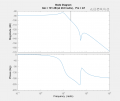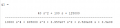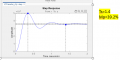# Controller for infinite phase margin

#### diegohelpme

Joined Jan 7, 2022
2
Hello, I have a transfer function of an active suspension system of a quarter of a vehicle, they ask me to calculate a controller from the Bode diagram that complies with overshoot of 33% and settling time 1. I have the problem that the margin of phase gives infinity, if someone could guide me I would appreciate it. Another question is because when graphing it, it gives me a curve of 10 ^ -5. Is this normal for active suspension systems?#### LvW

Joined Jun 13, 2013
1,586
I suppose your graph should represent the loop gain (magnitude and phase) - correct?
This display of the frequency-dependent gain of the open loop is necessary for finding the stability margin.
As I can see, your loop gain phase starts at zero deg.
This is an indication for a wrong loop gain simulation - it always must start at -180deg (DC negative feedback for a stable quiescent point). It seems that you have forgotten to inlclude the phase inversion (-180deg) at the summing node for input and DC feedback signal)

#### diegohelpme

Joined Jan 7, 2022
2
I suppose your graph should represent the loop gain (magnitude and phase) - correct?
This display of the frequency-dependent gain of the open loop is necessary for finding the stability margin.
As I can see, your loop gain phase starts at zero deg.
This is an indication for a wrong loop gain simulation - it always must start at -180deg (DC negative feedback for a stable quiescent point). It seems that you have forgotten to inlclude the phase inversion (-180deg) at the summing node for input and DC feedback signal)
How can I reverse the phase? .
Yes, the Bode plot represents the gain and the phase. What I did to get the diagram was to enter the system transfer function in matlab using the sisotool command.
If it is possible to contact you by mail I would appreciate it

#### LvW

Joined Jun 13, 2013
1,586
How can I reverse the phase? .
Yes, the Bode plot represents the gain and the phase. What I did to get the diagram was to enter the system transfer function in matlab using the sisotool command.
If it is possible to contact you by mail I would appreciate it